Are you stuck studying and need help with the subject of "quadratic functions"? In this article we will explain everything to you on the following topics:

Start by watching Daniel's introductory video on parables and quadratic functions!

Parabolas, Quadratic Functions, Overview, Vertex, Compression, Extension | Math by Daniel Jung

Math study book for the 5th to 10th grade

14,99€

The graph of quadratic functions is always a parabola. At the beginning we want to look at the so-called normal parabola \$ f \ left (x \ right) = x ^ 2 \$: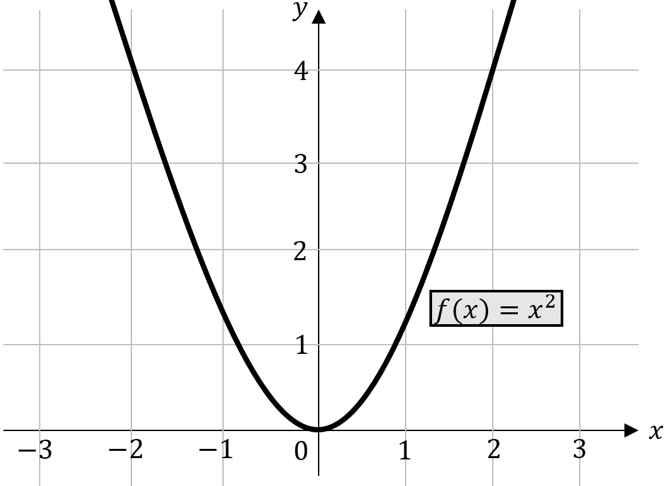We see that our normal parabola has its vertex at point \$ (0 | 0) \$. The vertex is the lowest or highest point of a parabola. We can transform (change or manipulate) this normal parabola in different ways. This means that we:

• move their vertex in the \$ x \$ direction (left or right) or
• move in \$ y \$ direction (up or down)
• We can also stretch it (make it narrower) or compress it (make it wider) or
• we can mirror them about the \$ x \$ axis so that their opening is facing down.

### Shift in \$ x \$ direction

We can consider the shift in the \$ x \$ direction in our function equation as follows. To do this, we take a look at the following coordinate system.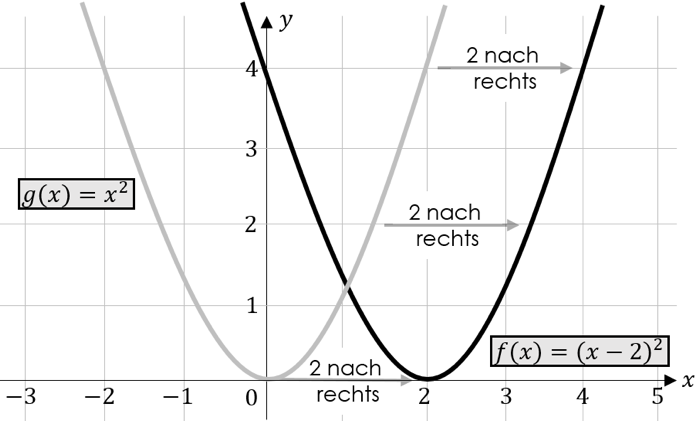The vertex of this parabola and all other points were shifted \$ 2 \$ units to the right based on the normal parabola (here: \$ g \ left (x \ right) = x ^ 2 \$).
If we take a look at the function equation, we see that it reads as follows:

\ [f \ left (x \ right) = {(x-2)} ^ 2 \]
A shift in the \$ x \$ direction can always be recognized by the fact that the value by which the parabola was shifted appears with the opposite sign in the brackets.

We also want to look at a parabola that has been shifted to the left. The functional equation of this parabola is:
\ [f \ left (x \ right) = {(x + 2)} ^ 2 \]

The parabola has been shifted to the left by \$ 2 \$ units. We recognize this from the fact that the \$ -2 \$ in our equation appears with an opposite sign within the brackets.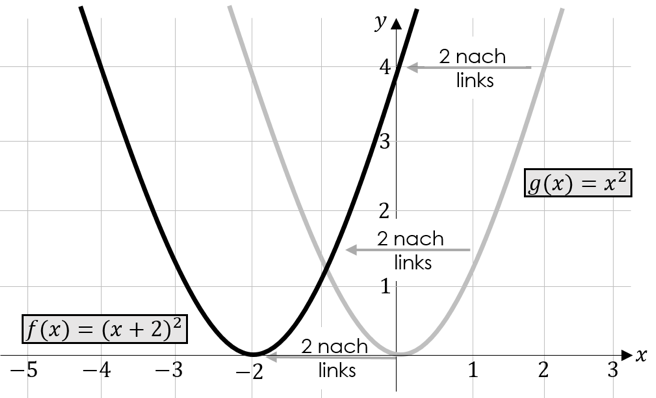Maths explained simply! Our learning book for the 5th to 10th grade

14,99€

### Shift in \$ y \$ direction

You can recognize the shift in the \$ y \$ direction by the fact that the value by which the parabola was shifted in the \$ y \$ direction is appended with the correct sign without brackets.

Let us consider the parabola below. This parabola has been shifted up by \$ 2 \$ units.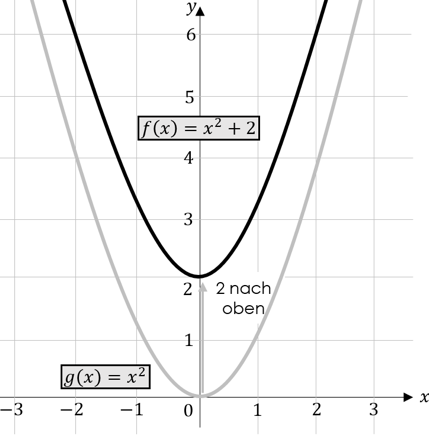The associated function equation must therefore be \$ f \ left (x \ right) = x ^ 2 + 2 \$.

Next we want to look at a parabola that has been moved down.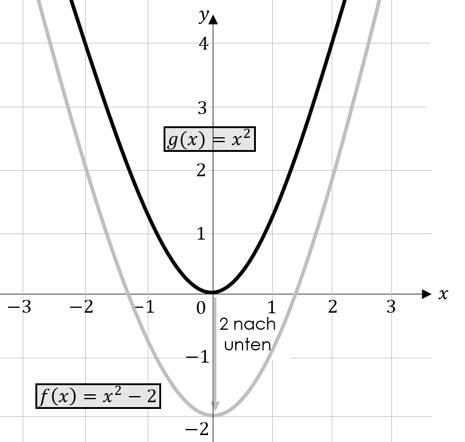We recognize from our function equation \$ f \ left (x \ right) = x ^ 2-2 \$ that our parabola has been shifted downwards.
Of course, it is also possible to carry out both a shift in the \$ x \$ direction and a shift in the \$ y \$ direction at the same time. For this we consider the following parabola.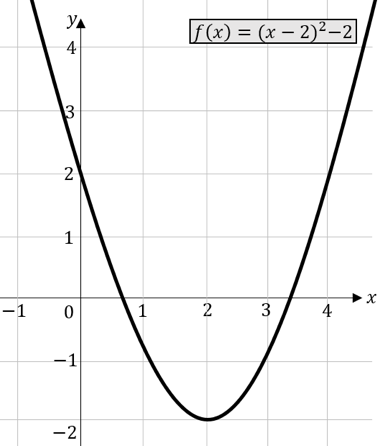This parabola has been shifted \$ 2 \$ units to the right and \$ 2 \$ units down. The function equation is: \$ f \ left (x \ right) = {(x-2)} ^ 2-2 \$
In the bracket we can see the shift by \$ 2 \$ units to the right and behind the bracket we see the shift by \$ 2 \$ units down. A functional equation which is given in the above form is called the vertex shape, since it is possible to read off the coordinates of the vertex directly. In our case \$ S (2 | -2) \$.

### Elongation / compression

If we want to stretch or compress a parabola, we have to multiply the functions by a factor \$ a \$.
\$ F \ left (x \ right) = x ^ 2 \$ then becomes \$ f \ left (x \ right) = a \ cdot x ^ 2 \$. The following rules apply:

The factor \$ a \$ indicates whether it is a stretch or a compression. The factor \$ a \$ is either directly in front of the \$ x ^ 2 \$ or, if our function equation is in the vertex form, directly in front of the bracket. To this end, let us briefly keep the following facts in mind.
The normal parabola \$ f \ left (x \ right) = x ^ 2 \$ has the factor \$ a = 1 \$. However, for reasons of mathematical laziness, we do not write these down. The normal parabola is neither stretched nor compressed.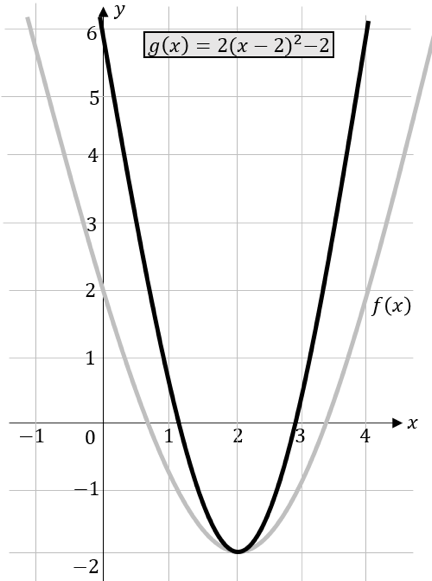Likewise, our parabola with the function equation \$ f \ left (x \ right) = {(x-2)} ^ 2-2 \$ is neither stretched nor compressed, since the factor \$ a \$ directly in front of the brackets also has the value \$ a = Has \$ 1.

A stretched parabola could have the following equation:

\ [g \ left (x \ right) = 2 {(x-2)} ^ 2-2 \]

We see that our factor \$ a \$ is now \$ a = 2 \$. Since \$ a \$ is greater than 1, the parabola would have to be stretched. For this we consider the functions \$ f (x) = {\ left (x-2 \ right)} ^ 2-2 \$ and \$ g (x) \$ in the adjacent figure. We see that our parabola \$ g \$ looks much narrower in relation to the parabola \$ f \$. So it is stretched.

In contrast, we want to look at a compressed parabola. We consider the following illustration.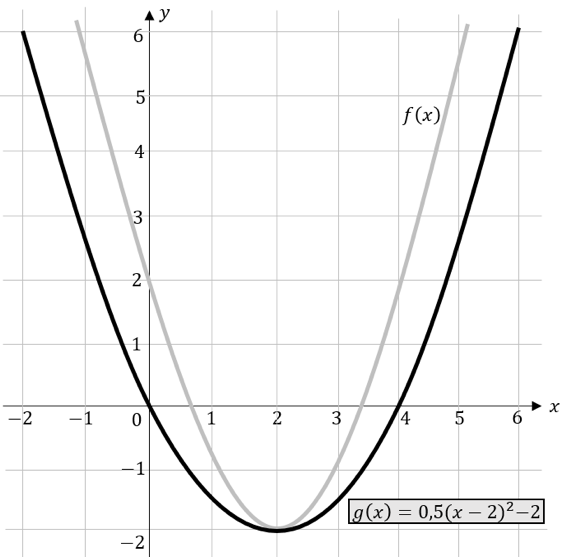The functional equations of our two parabolas are:

\ [f \ left (x \ right) = {(x-2)} ^ 2-2 \] and \ [g \ left (x \ right) = 0.5 {(x-2)} ^ 2-2 \]

We see that our parabola \$ g \$ is wider than our parabola \$ f \$. So it is compressed.

### Reflection on the \$ x \$ axis

We recognize a parabola mirrored on the \$ x \$ axis (opened downwards) by the fact that the factor \$ a \$ is negative. To do this, we consider the following representation: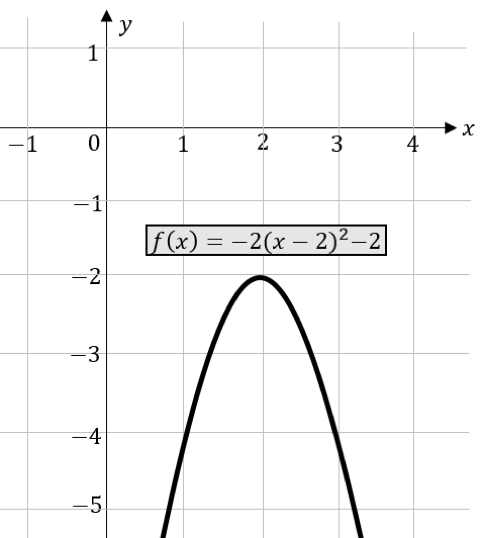The functional equation of our parabola is \$ f \ left (x \ right) = - 2 {\ left (x-2 \ right)} ^ 2-2 \$. The factor \$ a \$ has the value \$ a = -2 \$, so it is negative. Overall, the following transformations were carried out on this parabola:

### Zeroing a parabola

The zeros (points of intersection with the -axis) can be found using the procedures mentioned in the previous chapters. Which method is most suitable naturally depends on the form in which the functional equation is given. Please make it clear to yourself at this point that a parabola has either two zeros, one or even no zero.

Daniel explains the zeros of a parabola again in the tutorial video.

Math study book for the 5th to 10th grade

14,99€

### General shape and vertex shape

The functional equations of parabolas can exist in two different forms:

General form: \$ f \ left (x \ right) = a \ times x ^ 2 + b \ times x + c \$
Vertex shape: \$ f \ left (x \ right) = a \ cdot {\ left (x-d \ right)} ^ 2 + e \$

One can convert the general shape into the vertex shape and the vertex shape into the general shape.

Check out the tutorial on how to recognize a vertex!

Recognize vertex in slow, quadratic functions, parabolas | Math by Daniel Jung

Maths study book for the 5th to 10th grade

14,99€

### General shape in vertex shape

We assume that we have the following functional equation. In the first step, we factor out the factor \$ a \$ in front of the \$ x ^ 2 \$ (here the 2) and get:

\ [f \ left (x \ right) = 2x ^ 2 + 4x-3 = 2 (x ^ 2 + 2x-1,5) \]

Next we apply the first binomial formula to the first three summands and get:

\ [f \ left (x \ right) = 2 [{\ left (x + 1 \ right)} ^ 2-2.5] \]

Now we multiply the factor \$ a \$ by the constant number at the end of the function equation:

\ [f \ left (x \ right) = 2 {(x + 1)} ^ 2-5 \]

Now we can read off the coordinates of our vertex again: \$ S \ (-1 | -5) \$.

Recognize vertex shape / vertex shape with quadratic functions | Math by Daniel Jung

Maths study book for the 5th to 10th grade

14,99€

### Convert vertex shape to general shape

We assume that we have the following functional equation:

\ [f \ left (x \ right) = 3 {(x-2)} ^ 2 + 5 \]

First we apply the second binomial formula to solve the bracket. Remember that the term resulting from using the binomial formula must be put in parentheses.

\ [f \ left (x \ right) = 3 \ left (x ^ 2-4x + 4 \ right) +5 \]

Then the expression in brackets is multiplied by the factor \$ a \$ (here 3).

\ [f \ left (x \ right) = 3x ^ 2-12x + 12 + 5 \]

Finally we summarize and get our function equation in general form:

\ [f \ left (x \ right) = 3x ^ 2-12x + 17 \]

The graph below of the function \$ f (x) = - 0.025x ^ 2 + 2x \$ describes the trajectory of a golf ball after the tee. The axis indicates the horizontal distance from the tee point, the axis the height of the golf ball.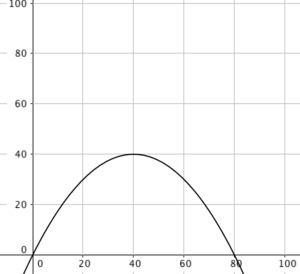1. What is the maximum height of the golf ball on its trajectory?
2. At what height is the golf ball at a horizontal distance of 20m?
3. How far is the golf ball landing point from the tee point?

Solution:

Exercise part 1:

The maximum height can be recognized directly from the? -Coordinate of the vertex when the parabolas open downwards. The vertex of our parabola has the coordinates \$ S (40 | 40) \$. The maximum height of our golf ball is therefore \$ 40? \$.

Exercise part 2:

In the first part of the exercise it was already described that the respective? -Coordinates indicate the height of the golf ball. We are now looking for the level above the \$ 20? \$ Mark. This cannot be read directly from the graph of the function. Therefore, we now need our function specification and insert the value \$ 20 \$. We obtain:

\ begin {align *}
?(20)=−0,025∙20^2+2∙20=30
\ end {align *}

The golf ball reaches a height of \$ 30? \$ Above the \$ 20? \$ Mark

Exercise part 3:

The landing point of the golf ball is represented by the right zero of our graph. This can be read off here without any problems. Our right zero has the coordinates \$? _ 2 (80 | 0) \$. So the distance to the tee point is exactly \$ 80? \$.

At this point we will deal with an alternative solution if the task forces you to determine it mathematically. First we set our function rule equal to 0 and get:

\ begin {align *}
−0,025∙?^2+2∙?=0
\ end {align *}

Do we now solve this equation? by first excluding a \$? \$:

\ begin {align *}
?∙(−0,025∙?+2)=0
\ end {align *}

Now we can apply the zero product theorem and get:

\ begin {align *}
? = 0 \ vee −0.025 ∙? + 2 = 0
\ end {align *}

So we immediately get our left zero, namely at the point \$ 0 \$ in the origin. We get our right zero by solving the remaining equation for \$? \$:

\ begin {align *}
\ begin {array} {rrcll} & −0.025 \ cdot x + 2 & = & 0 & | - 2 \
\ Leftrightarrow & −0.025 \ cdot x & = & -2 & |: (- 0.025) \
\ Leftrightarrow & x & = & 80 & \ end {array}
\ end {align *}

We get our expected result and have shown that the landing point 80? away from the tee point.

Daniel shows you how to bring the vertex shape into the general shape.

Vertex form to normal form by multiplying, parabolas, quadratic fct. | Math by Daniel Jung

Math study book for the 5th to 10th grade

14,99€